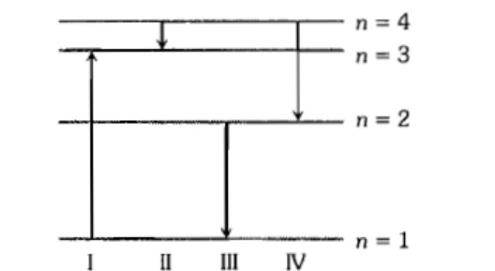The diagram shows the energy levels for an electron in a certain atom. Which transition shown represents the emission of a photon with the most energy?(1) I               (2) II                (3) III             (4) IV

Concept Questions :-

Spectral series
High Yielding Test Series + Question Bank - NEET 2020

Difficulty Level:

The kinetic energy of the electron in an orbit of radius r in the hydrogen atom is (e = electronic charge)

(1) $\frac{k{e}^{2}}{{r}^{2}}$        (2) $\frac{k{e}^{2}}{2r}$         (3) $\frac{k{e}^{2}}{r}$            (4) $\frac{k{e}^{2}}{2{r}^{2}}$

Concept Questions :-

Bohr's model of atom
High Yielding Test Series + Question Bank - NEET 2020

Difficulty Level:

Whenever a hydrogen atom emits a photon in the Balmer series:

(1) It may emit another photon in the Balmer series

(2) It must emit another photon in the Lyman series

(3) It may emit another photon in the Paschen series

(4) It need not emit any more photon

Concept Questions :-

Spectral series
High Yielding Test Series + Question Bank - NEET 2020

Difficulty Level:

The ionisation potential of hydrogen atom is

(1) 13.60 volt                (2) 8.24 volt

(3) 10.36 volt                (4) 14.24 bolt

Concept Questions :-

Bohr's model of atom
High Yielding Test Series + Question Bank - NEET 2020

Difficulty Level:

Which source is associated with a line emission spectrum?

(1) Electric fibre                   (2) Neon street Sign

(3) Red traffic light               (4) Sun

Concept Questions :-

Spectral series
High Yielding Test Series + Question Bank - NEET 2020

Difficulty Level:

Ionisation potential of hydrogen atom is 13.6 eV. Hydrogen atoms in the ground state are excited by monochromatic radiation of photon energy 12.1 eV. According to Bohr's theory, the spectral lines emitted by hydrogen will be:

(1) two          (2) three         (3) four             (4) one

Concept Questions :-

Spectral series
High Yielding Test Series + Question Bank - NEET 2020

Difficulty Level:

Frequency of the series limit of Balmer series of hydrogen atom in terms of Rydberg constant R and velocity of light C is:

(1) $\frac{RC}{4}$           (2) RC            (3) $\frac{4}{RC}$            (4) 4RC

Concept Questions :-

Spectral series
High Yielding Test Series + Question Bank - NEET 2020

Difficulty Level:

Orbital acceleration of electron is

(1) $\frac{{n}^{2}{h}^{2}}{4{\mathrm{\pi }}^{2}{\mathrm{m}}^{2}{\mathrm{r}}^{3}}$                  (2) $\frac{{n}^{2}{h}^{2}}{4{n}^{2}{\mathrm{r}}^{3}}$

(3) $\frac{4{n}^{2}{h}^{2}}{{\mathrm{\pi }}^{2}{\mathrm{m}}^{2}{\mathrm{r}}^{3}}$                     (4) $\frac{4{n}^{2}{h}^{2}}{4{\mathrm{\pi }}^{2}{\mathrm{m}}^{2}{\mathrm{r}}^{3}}$

Concept Questions :-

Bohr's model of atom
High Yielding Test Series + Question Bank - NEET 2020

Difficulty Level:

Consider the spectral line resulting from transition n = 2 to n = 1 in the atoms and ions given below.  The shorterst wavelength is given by

(1) Hydrogen atom

(2) Deuterium

(3) Singly ionised helium

(4) Doubly ionised lithium

Concept Questions :-

Spectral series
High Yielding Test Series + Question Bank - NEET 2020

Difficulty Level:

The valence electron in alkali metal is a 

(1) f-electron       (2) p-electron         (3) s-electron         (4) d-electron

Concept Questions :-

Various atomic model